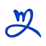# 2023年JS代码怎么出高大上的代码dearweb 发布：2023-11-10 12:26:12阅读：

```// 使用箭头函数
const add = (a, b) => a + b;

// 使用解构赋值
const { firstName, lastName } = person;

// 使用模板字符串
const greeting = `Hello, \${firstName} \${lastName}!`;```

```// 导入模块
import { func1, func2 } from './myModule';

// 导出模块
export function myFunction() {
// 代码逻辑
}```

```// 使用Promise
fetchData()
.then(data => {
// 处理数据
})
.catch(error => {
// 处理错误
});

// 使用async/await
async function fetchData() {
try {
const response = await fetch('https://api.example.com/data');
const data = await response.json();
// 处理数据
} catch (error) {
// 处理错误
}
}```

```// 函数式编程
const numbers = [1, 2, 3, 4, 5];

const doubled = numbers.map(n => n * 2);

const sum = doubled.reduce((acc, curr) => acc + curr, 0);```

```// 使用React
import React, { useState } from 'react';

function MyComponent() {
const [count, setCount] = useState(0);

return (
<div>
<p>Count: {count}</p>
<button onClick={() => setCount(count + 1)}>Increment</button>
</div>
);
}```

24人点赞 返回栏目 提问